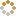﻿ Ningbo University of Technology Online Judge ::  Max Sum
• ###  Max Sum

• 时间限制: 1000 ms　内存限制: 65535 K
• 问题描述
• Given a sequence a,a,a......a[n], your job is to calculate the max sum of a sub-sequence. For example, given (6,-1,5,4,-7), the max sum in this sequence is 6 + (-1) + 5 + 4 = 14.
• 输入
• The first line of the input contains an integer T(1<=T<=20) which means the number of test cases. Then T lines follow, each line starts with a number N(1<=N<=100000), then N integers followed(all the integers are between -1000 and 1000).
• 输出
• For each test case, you should output two lines. The first line is "Case #:", # means the number of the test case. The second line contains three integers, the Max Sum in the sequence, the start position of the sub-sequence, the end position of the sub-sequence. If there are more than one result, output the first one. Output a blank line between two cases.
• 样例输入
• ```2
5 6 -1 5 4 -7
7 0 6 -1 1 -6 7 -5```
• 样例输出
• ```Case 1:
14 1 4

Case 2:
7 1 6
```
• 提示
• `无`
• 来源
• `Ignatius.L`
• 操作
﻿